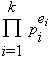# number of divisors

The number of positive divisors of n is denoted by d(n) (or tau(n) or better, τ(n). Here are the first few values of this function:

 integer n d(n) 1 2 3 4 5 6 7 8 9 10 11 12 13 14 15 16 1 2 2 3 2 4 2 4 3 4 2 6 2 4 4 5

Clearly, for primes p, d(p)=2; and for prime powers, d(pn)=n+1. For example, 34 has the five (4+1) positive divisors 1, 3, 32, 33, and 34.

Since d(x) is a multiplicative function, this is enough to know d(n) for all integers n--if the canonical factorization of n isthen the number of divisors is

τ(n) = (e1+1)(e2+1)(e3+1) ... (ek+1).

For example, 4200 is 23315271, so it has (3+1)(1+1)(2+1)(1+1) = 48 positive divisors.